# QUESTION3 25 marks (allow 30 minutes) The Figure below (Figure 3) shows a pipe system with a valve and two reservoirs. A pump transports a constant flow rate of Q 0.1 m3/s of water from reservoi...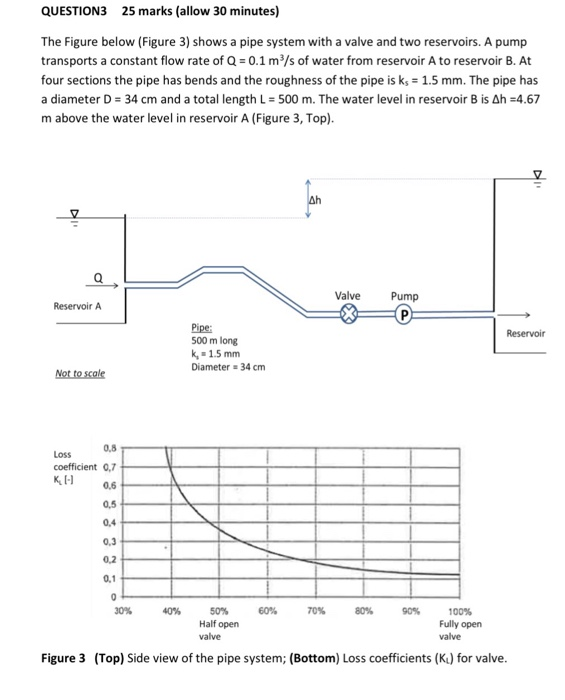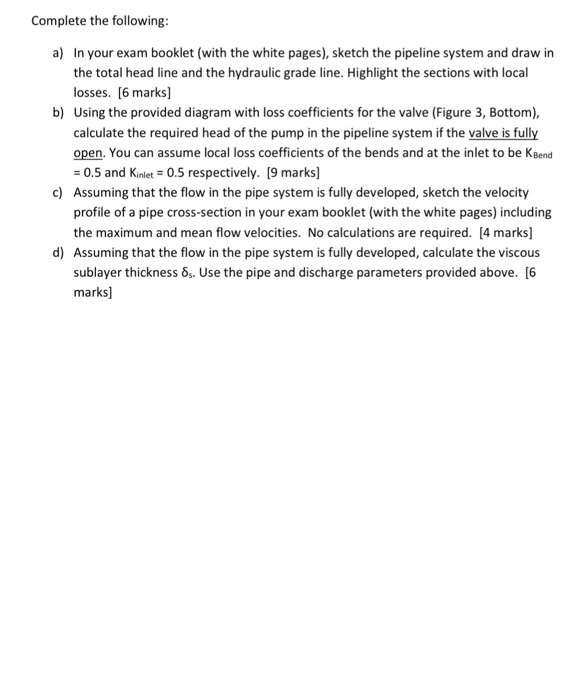QUESTION3 25 marks (allow 30 minutes) The Figure below (Figure 3) shows a pipe system with a valve and two reservoirs. A pump transports a constant flow rate of Q 0.1 m3/s of water from reservoir A to reservoir B. At four sections the pipe has bends and the roughness of the pipe is ks 1.5 mm. The pipe has a diameter D 34 cm and a total length L = 500 m. The water level in reservoir B is Δh-4.67 m above the water level in reservoir A (Figure 3, Top) Valve Pump Reservoir A Pipe 500 m long k, 1.5 mm Diameter 34 cm 0.8 coefficient 0,7 K 0.6 Loss 0.5 0,3 0.2 70% 8% 60% 50% Half open 90% 100% Fully open Figure 3 (Top) Side view of the pipe system; (Bottom) Loss coefficients (Kt) for valve
Complete the following a) In your exam booklet (with the white pages), sketch the pipeline system and draw in the total head line and the hydraulic grade line. Highlight the sections with local losses. [6 marks] Using the provided diagram with loss coefficients for the valve (Figure 3, Bottom), calculate the required head of the pump in the pipeline system if the valve is fully open. You can assume local loss coefficients of the bends and at the inlet to be KBend 0.5 and Kinlet 0.5 respectively. [9 marks] Assuming that the flow in the pipe system is fully developed, sketch the velocity profile of a pipe cross-section in your exam booklet (with the white pages) including the maximum and mean flow velocities. No calculations are required. [4 marks b) c) d Assuming that the flow in the pipe system is fully developed, calculate the viscous 8. Use the pipe and discharge parameters provided above. (6 sublayer thickness marks]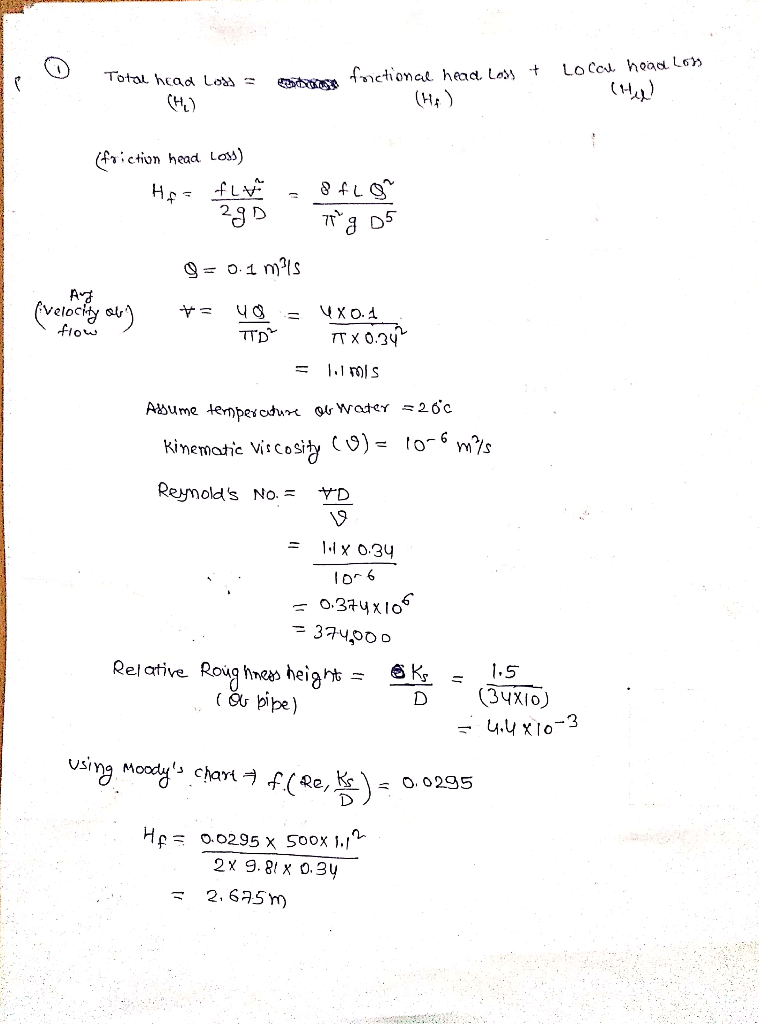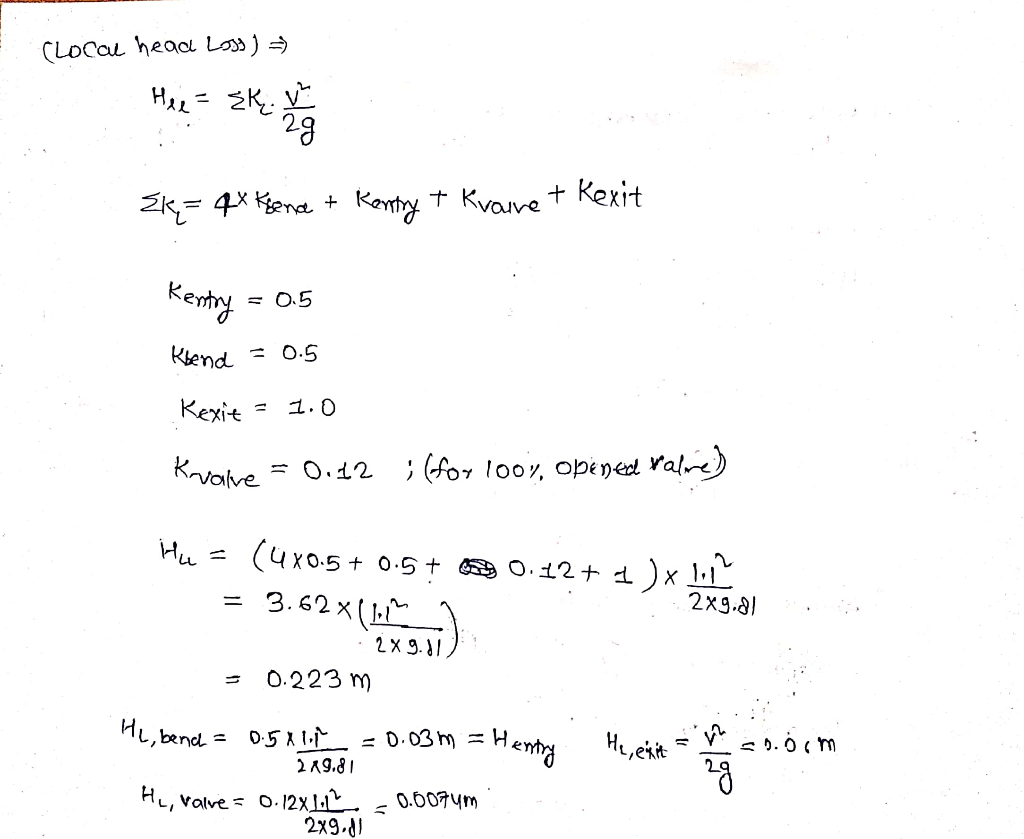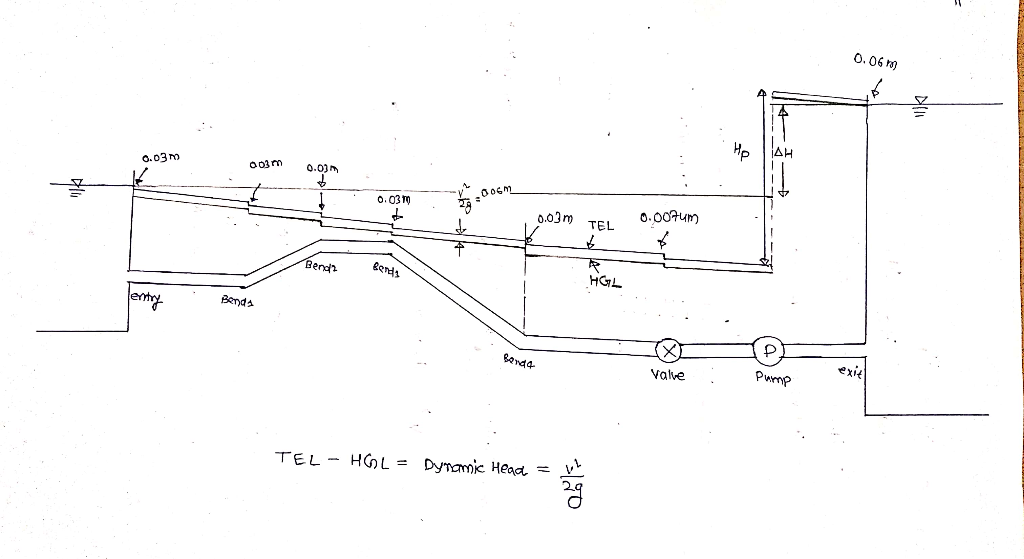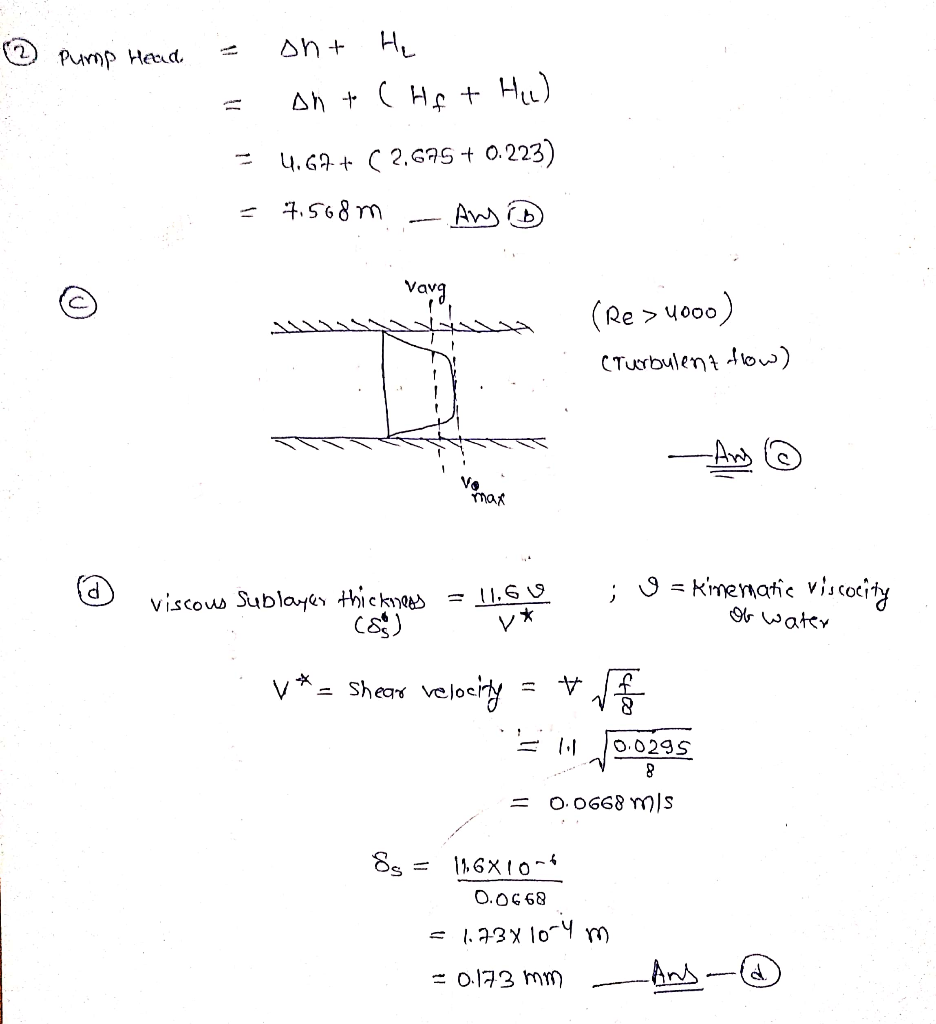#### Earn Coin

Coins can be redeemed for fabulous gifts.

Similar Homework Help Questions
• ### 25 marks (allow 30 minutes) QUESTION3 The Figure below (Figure 3) shows a pipe system with a valve and two reservoirs. A pump transports a constant flow rate of Q # 0.1 mls of water from reser...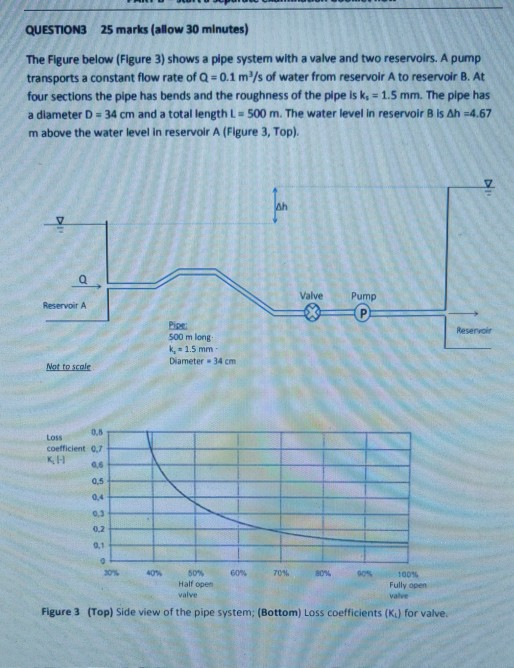25 marks (allow 30 minutes) QUESTION3 The Figure below (Figure 3) shows a pipe system with a valve and two reservoirs. A pump transports a constant flow rate of Q # 0.1 mls of water from reservoir A to reservoir B. At four sections the pipe has bends and the roughness of the pipe is k, 1.5 mm. The pipe has a diameter D 34 cm and a total length L 500 m. The water level in reservoir B is...

• ### Water (density 998 kg/mº, dynamic viscosity 0.001 Pa s) is pumped between two reservoirs at a...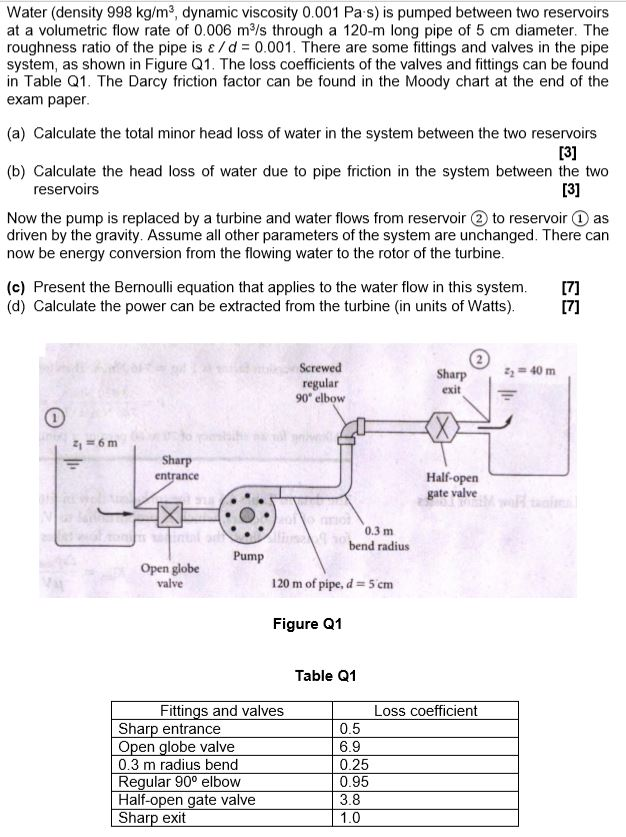Water (density 998 kg/mº, dynamic viscosity 0.001 Pa s) is pumped between two reservoirs at a volumetric flow rate of 0.006 m/s through a 120-m long pipe of 5 cm diameter. The roughness ratio of the pipe is a/d = 0.001. There are some fittings and valves in the pipe system, as shown in Figure Q1. The loss coefficients of the valves and fittings can be found in Table Q1. The Darcy friction factor can be found in the Moody...

• ### Problem 3 A pipeline delivers water from Reservoir 1 to Reservoir 2 as shown in the following figure. The water levels at Reservoirs 1 and 2 are 50 ft and 20 ft, respectively. A globe valve is in...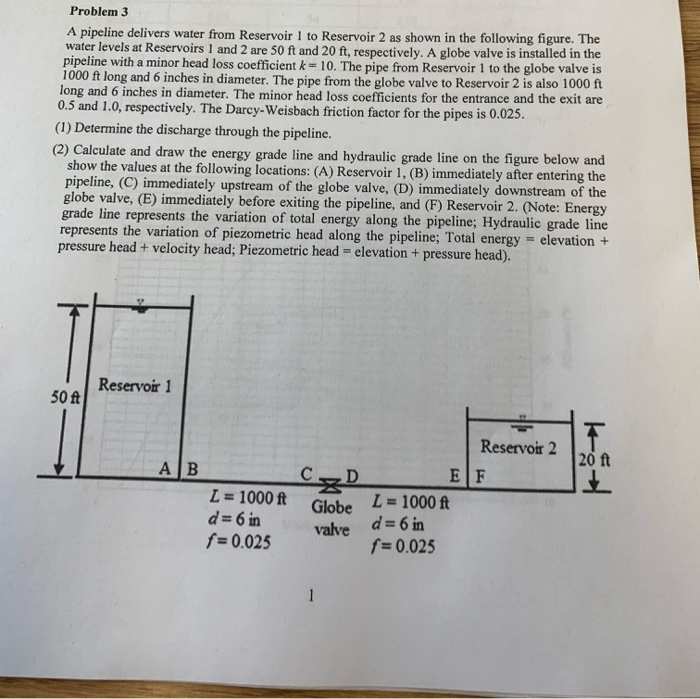Problem 3 A pipeline delivers water from Reservoir 1 to Reservoir 2 as shown in the following figure. The water levels at Reservoirs 1 and 2 are 50 ft and 20 ft, respectively. A globe valve is installed in the pipeline with a minor head loss coefficient k 10. The pipe from Reservoir 1 to the globe valve is 1000 ft long and 6 inches in diameter. The pipe from the globe valve to Reservoir 2 is also 1000 ft...

• ### A pump transports water from Tank 1 to Tank 2 through a constant-diameter piping system as...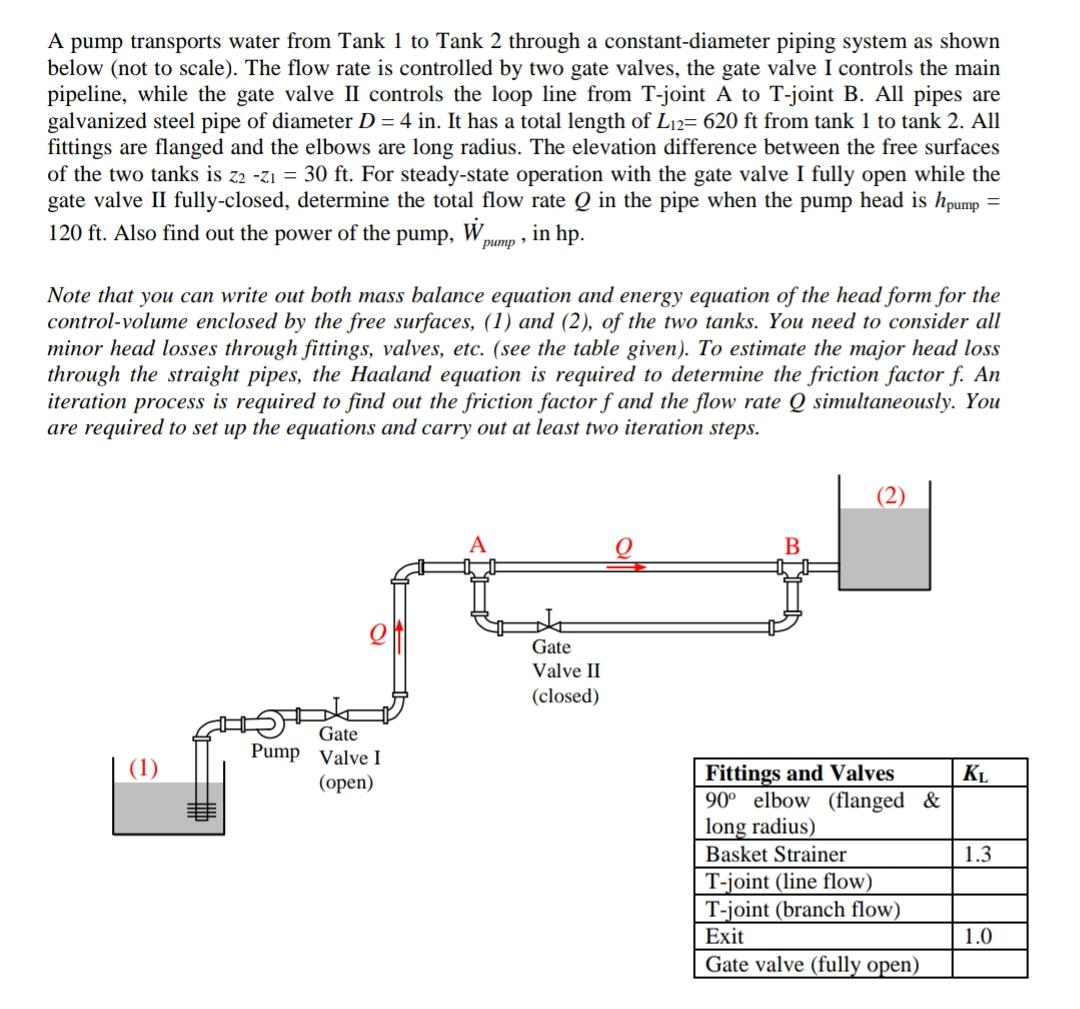A pump transports water from Tank 1 to Tank 2 through a constant-diameter piping system as shown below (not to scale). The flow rate is controlled by two gate valves, the gate valve I controls the main pipeline, while the gate valve II controls the loop line from T-joint A to T-joint B. All pipes are galvanized steel pipe of diameter D = 4 in. It has a total length of Li2= 620 ft from tank 1 to tank 2....

• ### The figure below shows a condenser/cooling tower pipe system needed to remove heat from a water...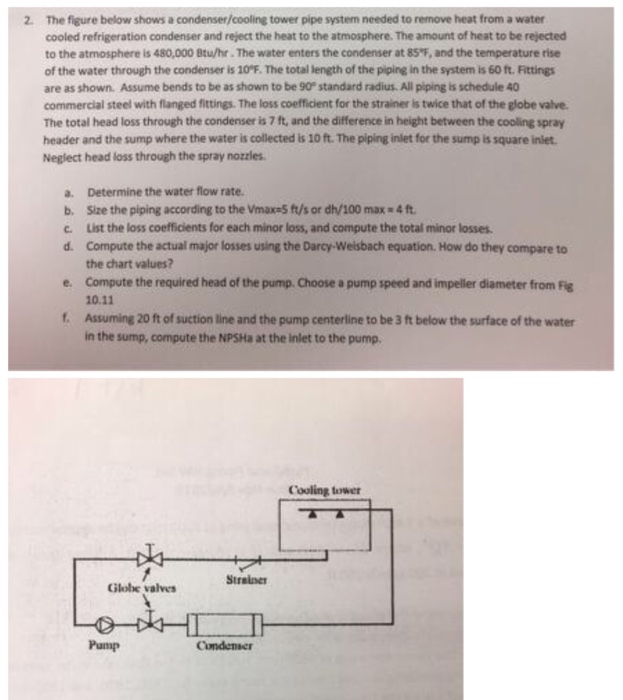The figure below shows a condenser/cooling tower pipe system needed to remove heat from a water cooled refrigeration condenser and reject the heat to the atmosphere. The amount of heat to be rejected to the atmosphere is 480,000 Btu/hr. The water enters the condenser at 85"F, and the temperature rise of the water through the condenser is 10°F. The total length of the piping in the system is 60 ft. Fittings are as shown. Assume bends to be as shown...

• ### (100 points) Water at 25°C is pumped at a rate of 0.075m's from a reservoir 20m above a pump to a free 1....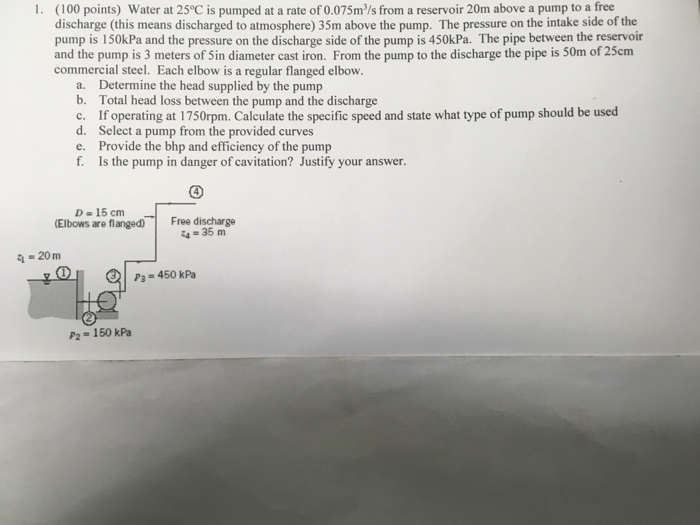(100 points) Water at 25°C is pumped at a rate of 0.075m's from a reservoir 20m above a pump to a free 1. discharge (this means discharged to atmosphere) 35m above the pump. The pressure on the intake side of the pump is 150kPa and the pressure on the discharge side of the pump is 450kPa. The pipe between the reservoir and the pump is 3 meters of Sin diameter cast iron. From the pump to the discharge the pipe...

• ### QUESTION 9 15 marks Water is pumped at a constant rate of SOL/s through the system...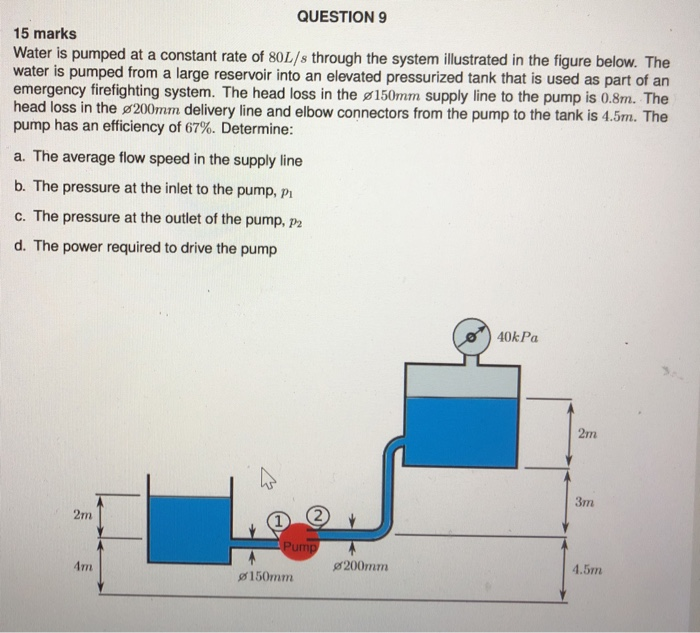QUESTION 9 15 marks Water is pumped at a constant rate of SOL/s through the system illustrated in the figure below. The water is pumped from a large reservoir into an elevated pressurized tank that is used as part of an emergency firefighting system. The head loss in the 150mm supply line to the pump is 0.8m. The head loss in the s200mm delivery line and elbow connectors from the pump to the tank is 4.5m. The pump has an...

• ### Problem Statement 1 The pump in the system shown below is used to transport water from...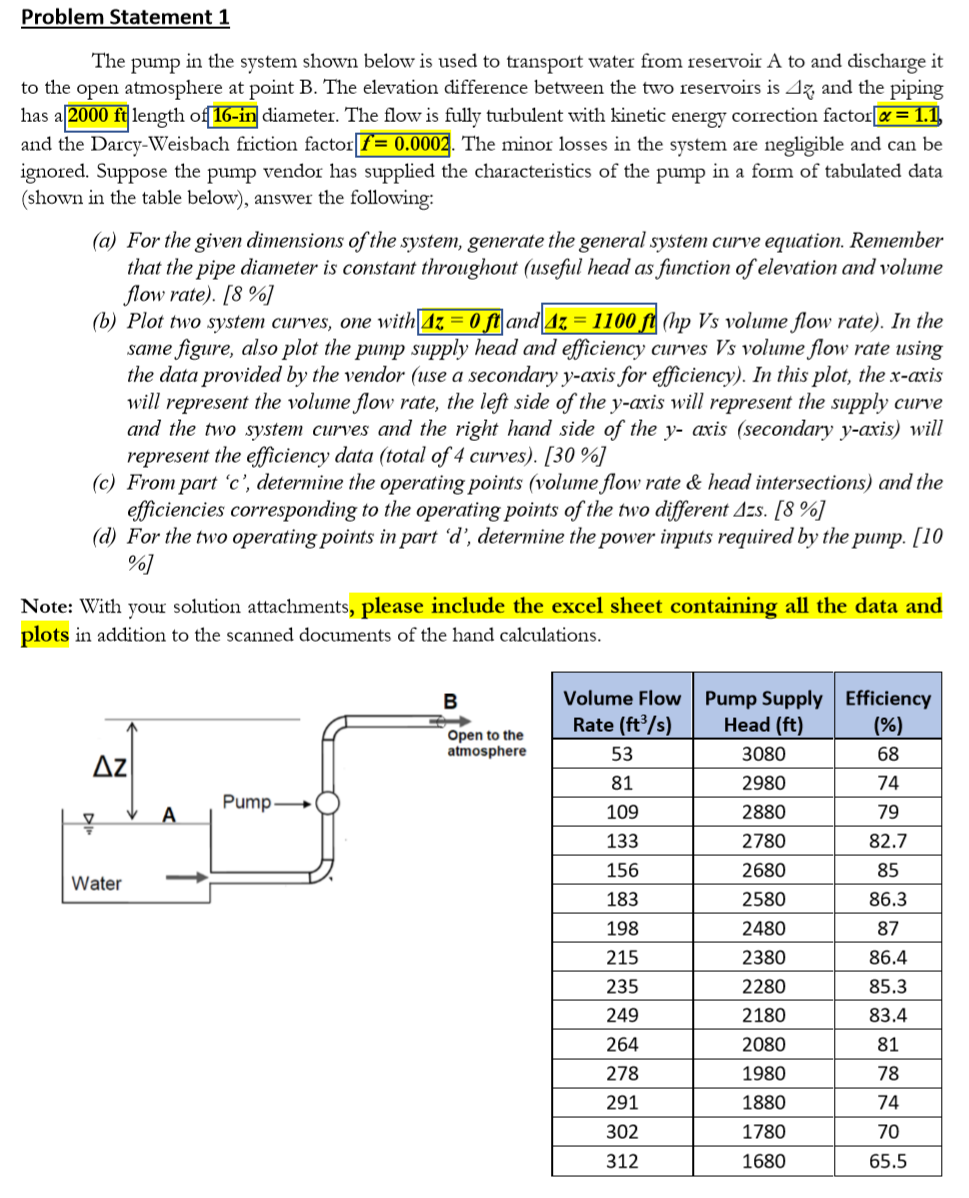Problem Statement 1 The pump in the system shown below is used to transport water from reservoir A to and discharge it to the open atmosphere at point B. The elevation difference between the two reservoirs is Az, and the piping has a 2000 ft length of 16-in diameter. The flow is fully turbulent with kinetic energy correction factor = 1.1 and the Darcy-Weisbach friction factor f = 0.0002. The minor losses in the system are negligible and can be...